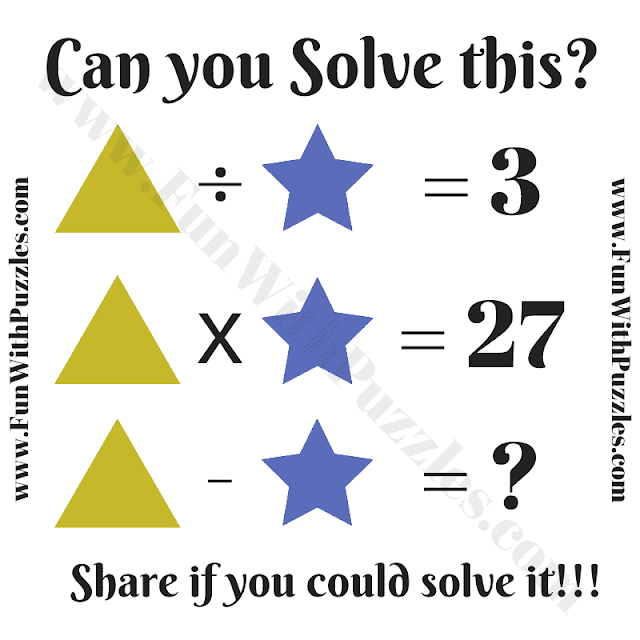Sharpen your mathematical skills with this intriguing picture math number puzzle. Replace variables with geometrical symbols and crack the equations to unveil the missing number.

Delving into Geometrical Algebra: Dive into the world of geometrical algebra with this captivating number puzzle. It's a unique twist on traditional equations, where you'll replace variables with geometric symbols. This puzzle combines math with creative problem-solving, offering a stimulating challenge for teens and math enthusiasts.Can you solve this Picture Maths Number Puzzle?

Cracking the Equations: You'll be presented with a series of mathematical equations where triangles and stars represent unknown values. To solve the puzzle, decipher the values of these geometrical symbols and use them to find the missing number in the final equation. It's a fantastic exercise for algebraic thinking and mathematical reasoning.

Mind-Boosting Math: This puzzle not only tests your mathematical prowess but also enhances your problem-solving skills. It encourages you to think creatively and logically to deduce the values of the geometric symbols. Whether you're a teen looking to improve your math skills or a math enthusiast seeking a fun challenge, this puzzle is perfect for you.

Join the Puzzle Fun: Are you up for the challenge? Solve the equations, unveil the secrets behind the geometric symbols, and triumph over this brain-teasing puzzle. Don't forget to share your answers and thoughts in the comments section.

The answer to this "Maths Puzzle Question for Students", can be viewed by clicking the answer button.

#### 1 comment:

bennyvenky52@gmail.com said...

9-3=6